Distribution Analyses

## QQ Ref Line

After choosing Curves:QQ Ref Line, you can use the QQ Ref Line dialog to add distribution reference lines to QQ plots.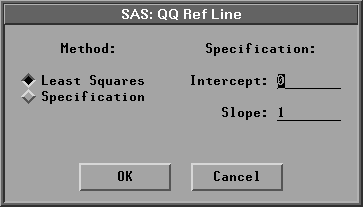Figure 38.35: QQ Ref Line Dialog

The default adds a least squares regression line. You can also specify your own reference line by choosing Method:Specification and specifying both the intercept and slope.

If you select a Weight variable, you can add a weighted least squares regression line to the normal QQ plot. If the data are normally distributed with mean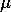and standard deviation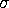and if each observation has approximately the same weight (w0), then the least squares regression line has approximately interceptand slopefor vardef=WDF/WEIGHT and slope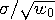for vardef=DF/N.

A normal QQ plot with a least squares reference line is shown in Figure 38.36. Use the sliders to change the intercept and slope of the reference line.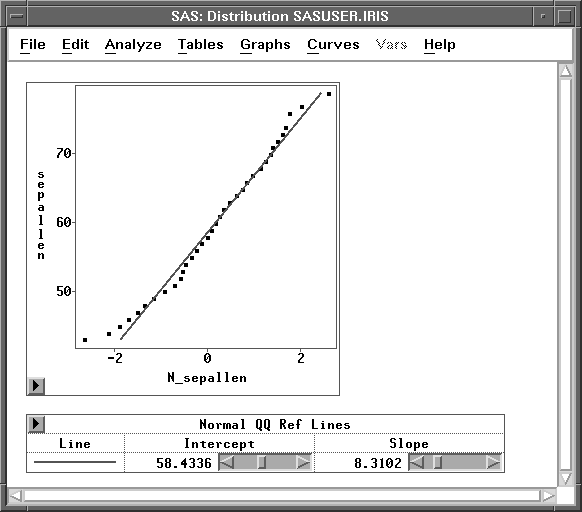Figure 38.36: Normal QQ Plot with a Reference Line

Copyright © 2007 by SAS Institute Inc., Cary, NC, USA. All rights reserved.